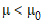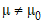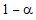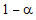# NMath User's Guide

41.1 Common Interface (.NET, C#, CSharp, VB, Visual Basic, F#)

All hypothesis test classes share substantially the same interface. Once you learn how to use one test, it's easy to use any of the others.

Static Properties

All hypothesis test classes have static DefaultAlpha properties that get and set the default alpha level associated with tests of that type. The default value is 0.01. For instance:

Code Example – C# hypothesis tests

```var test1 = new OneSampleTTest();
```
```// test1.Alpha == 0.01
```
```OneSampleTTest.DefaultAlpha = 0.05;
```
```var test2 = new OneSampleTTest();
```
```// test2.Alpha == 0.05
```

Code Example – VB hypothesis tests

```Dim Test1 As New OneSampleTTest()
```
```'' test1.Alpha == 0.01
```
```OneSampleTTest.DefaultAlpha = 0.05
```
```Dim Test2 As New OneSampleTTest()
```
```'' test2.Alpha == 0.05
```

Similarly, all hypothesis test classes have static DefaultType properties that get and set the default form of the alternative hypothesis. The form is specified using the enumeration, with the following enumerated values:

Left indicates a one-sided form to the left,.

Right indicates a one-sided form to the right,.

TwoSided indicates a two-sided form,.

The default value for all test classes is HypothesisType.TwoSided. For example:

Code Example – C# hypothesis tests

```var test1 = new OneSampleTTest();
```
```// test1.Type == HypothesisType.TwoSided
```
```OneSampleTTest.DefaultType = HypothesisType.Left;
```
```var test2 = new OneSampleTTest();
```
```// test2.Type == HypothesisType.Left
```

Code Example – VB hypothesis tests

```Dim Test1 As New OneSampleTTest()
```
```'' test1.Type == HypothesisType.TwoSided
```
```OneSampleTTest.DefaultType = HypothesisType.Left
```
```Dim Test2 As New OneSampleTTest()
```
```'' test2.Type == HypothesisType.Left
```

Creating Hypothesis Test Objects

All hypothesis test classes provide two paths for constructing instances of that type:

A parameter-based method, in which all necessary sample and population parameters are explicitly specified.

A data-based method, in which sample parameters are computed from supplied sample data.

NOTE—In the data-based method, once sample parameters have been computed from the given data, the data is discarded, and cannot be recovered from the test object.

For example, a one-sample z-test compares a single sample mean to an expected mean from a normal distribution with known standard deviation. This code constructs a OneSampleZTest object by explicitly specifying a sample mean, sample size, population mean, and population standard deviation:

Code Example – C# hypothesis tests

```double xbar = 112.8;
```
```int n = 9;
```
```double mu0 = 100;
```
```double sigma = 15;
```
```var test = new OneSampleZTest( xbar, n, mu0, sigma );
```

Code Example – VB hypothesis tests

```Dim XBar As Double = 112.8
```
```Dim N As Integer = 9
```
```Dim Mu0 As Double = 100
```
```Dim Sigma As Double = 15
```
```Dim Test As New OneSampleZTest(XBar, N, Mu0, Sigma)
```

This code constructs a OneSampleZTest object by supplying a vector of sample data, and the necessary population parameters:

Code Example – C# hypothesis tests

```var data =
```
```  new DoubleVector( "[ 116 110 111 113 112 113 111 109 121 ]" );
```
```double mu0 = 100;
```
```double sigma = 15;
```
```var test = new OneSampleZTest( data, mu0, sigma );
```

Code Example – VB hypothesis tests

```Dim MyData As New DoubleVector("[ 116 110 111 113 112 113 111 109
121 ]")
```
```Dim Mu0 As Double = 100
```
```Dim Sigma As Double = 15
```
```Dim Test As New OneSampleZTest(MyData, Mu0, Sigma)
```

In this case, the sample mean and sample size are calculated from the given data. The data-based method supports sample data in vectors, arrays, and data frame columns.

In both the parameter-based method and the data-based method, the alpha level for the hypothesis test is set to the current value specified by the static DefaultAlpha property, and the form of the hypothesis test is set to the current DefaultType, as described above.

Constructors are also provided for all test classes that enable you to set the alpha level and hypothesis type to non-default values. For example:

Code Example – C# hypothesis tests

```var test = new OneSampleZTest( data, mu0, sigma, 0.05,
```
```  HypothesisType.Left );
```

Code Example – VB hypothesis tests

```Dim Test As New OneSampleZTest(MyData, Mu0, Sigma, 0.05,
```
```  HypothesisType.Left)
```

Properties of Hypothesis Test Objects

All hypothesis test classes provide the following read-only properties:

Distribution gets the distribution of the test statistic associated with the hypothesis test.

Statistic gets the value of the test statistic associated with this hypothesis test.

P gets the p-value associated with the test statistic.

Reject tests whether the null hypothesis can be rejected, using the current hypothesis type and alpha level.

LeftCriticalValue gets the one-sided to the left critical value based on the current probability distribution and alpha level.

RightCriticalValue gets the one-sided to the right critical value based on the current probability distribution and alpha level.

LeftProbability gets the area under the probability distribution to the left of the test statistic.

RightProbability gets the area under the probability distribution to the right of the test statistic.

LowerConfidenceLimit gets thelower confidence limit for the true mean.

UpperConfidenceLimit gets theupper confidence limit for the true mean.

SEM gets the standard error of the mean.

The following read-write properties are also provided:

Alpha gets and sets the alpha level associated with the hypothesis test.

Type gets and sets the form of the alternative hypothesis associated with the hypothesis test.

Additionally, each hypothesis test provides properties for accessing the specific sample and population parameters that define the test. For example, a OneSampleZTest has additional properties for accessing the sample mean, Xbar, the sample size, N, the population mean, Mu0, and the population standard deviation, Sigma.

Modifying Hypothesis Test Objects

All hypothesis test classes provide Update() methods for modifying a test with new sample parameters or sample data, and new population parameters. For example, if test is a TwoSampleFTest instance, this code updates the test with two new samples, taken from two columns in a data frame df:

Code Example – C# hypothesis tests

```test.Update( df, df  );
```

Code Example – VB hypothesis tests

```Test.Update(DF(3), DF(7))
```

Printing Results

All hypothesis test classes provide a ToString() method that returns a formatted string representation of the test results. For instance:

Code Example – C# hypothesis tests

```var data1 = new DoubleVector( "9.21 11.51 12.79 11.85 9.97
```
```  8.79 9.69 9.68 9.19" );
```
```var data2 = new DoubleVector( "7.53 7.48 8.08 8.09 10.15
```
```  8.40 10.88 6.13 7.90 7.05 7.48 7.58 8.11" );
```
```var test = new TwoSampleFTest( data1, data2, 0.05,
```
```  HypothesisType.TwoSided );
```
```Console.WriteLine( test.ToString() );
```

Code Example – VB hypothesis tests

```Dim MyData1 As New DoubleVector("9.21 11.51 12.79 11.85 9.97 8.79
9.69 9.68 9.19")
```
```Dim MyData2 As New DoubleVector("7.53 7.48 8.08 8.09 10.15 8.40
10.88 6.13 7.90 7.05 7.48 7.58 8.11")
```
```Dim Test As New TwoSampleFTest(MyData1, MyData2, 0.05,
HypothesisType.TwoSided)
```
```Console.WriteLine(Test.ToString())
```

The output is:

```Two Sample F Test
```
```-----------------

```

```Sample Sizes = 9 and 13
```
```Standard Deviations = 1.39787139767736 and 1.23808008936914
```
```Variances = 1.95404444444444 and 1.53284230769231
```
```Ratio of Variances = 1.27478504125206
```
```Computed F statistic: 1.27478504125206, num df = 8, denom df = 12

```

```Hypothesis type: two-sided
```
```Null hypothesis: true ratio of variances = 1
```
```Alt hypothesis: true ratio of variances != 1
```
```P-value: 0.679745985376403
```
```RETAIN the null hypothesis for alpha = 0.05
```
```0.95 confidence interval: 0.363002872041806 5.3536732579205
```

Top

Top